Home > mini projects > Decoder and its Analysis

Decoder and its Analysis

Abstract - We review the main advantages in developing CMOS Decoder circuit. In addition to we describe its working principle. It can explain the advantages of basic Decoder and the thresholds of vatious parameters of circuit .Computer simulations show that the circuit has desirable desirable transfer characteristics than ones with resistors.We also compared our basic decoder circuit with the standard SN74LVC1G139 2-to-4 Line Decoder

# I. Introduction

Ideally the Decoder circuit was made to decode the encoded information and here as its name indicates, a decoder is a circuit component that decodes an input code. Given a binary code of n-bits, a decoder will tell which code is this out of the 2^n possible codes. Thus, a decoder has n-inputs and 2^n outputs. Each of the 2^n outputs corresponds to one of the possible 2^n input combinations. Thus, each output line equals 1 at only one input combination but is equal to 0 at all other combinations.Now considering a 2 to4 decoder which is a building block to design any input decoder block more than two input whose output of the decoder is actually a minterm resulting from a certain combination of the inputs, that is

D0 =A1' A0', ( minterm m0) which corresponds to input 00

D1 =A1' A0, ( minterm m1) which corresponds to input 01

D2 =A1 A0', ( minterm m2) which corresponds to input 10

D3 =A1 A0, ( minterm m3) which corresponds to input 11

## Applications:

Decoder applications many in the field of electonics and communication.Some of them are as follow

constuction of n Bit Decoder

BCD to decimal decoder

BCD to 7 segment decoder

Microprocessor memory systems

- Selecting different banks of memory

Microprocessor input/output systems

- Selecting different devices

Microprocessor instruction decoding

- Enabling different functional unit

Memory chips

- Enabling different row of memory depending on address

Solving the boolean expressions by additional gates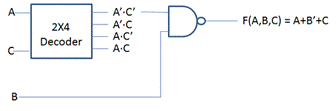## Specifications:

For the correct working of any any device specifications should be of great concern ,we cannot just despice them.Based on the model in Ngspice metal oxide semiconductors the circuit has good outputs when the voltage range from 4.0 v to 5v for logic 1 and 0v to 1v for logic 0 and input voltage VCC can be from 4.3v to 6.3v

## Netlist for the simulation of decoder circuit:

Code:

.subckt inverter 1 3 0 2

.model MOSFETN nmos

m1 2 1 0 0 MOSFETN

.model MOSFETP pmos

m2 2 1 3 3 MOSFETP

.ends inverter

Code:

.model MOSFETN nmos

m1 4 1 3 0 MOSFETN

m2 3 2 0 0 MOSFETN

m5 6 4 0 0 MOSFETN

.model MOSFETP pmos

m3 4 1 5 5 MOSFETP

m4 4 2 5 5 MOSFETP

m6 6 4 5 5 MOSFETP

.ends AND

Code:

*2-i/p DECODER

x1 1 5 0 3 inverter

x2 2 5 0 4 inverter

.include and.cir

x3 3 4 5 0 7 and

x4 3 2 5 0 8 and

x5 1 4 5 0 6 and

x6 1 2 5 0 9 and

vdd 5 0 dc 5

v1 1 0 pulse(0 5 0 0 0 25u 50u)

v2 2 0 pulse(0 5 0 0 0 50u 100u)

.tran 0.1u 200u

.control

run

display

Plot v(1)

Plot v(2)

plot v(7)

plot v(8)

plot v(6)

plot v(9)

.endc

.end

## Explanation:

In the netlist given as in the figure below we used one subcicuit to generate a simulated inverter using CMOS in Ngspice and another subcircuit for generation of a AND circuit and the connections made to obtain the outputs.

Let us discuss the operation and combinational circuit design of a decoder by taking the specific example of a 2-to-4 decoder. It contains two inputs denoted by A1 and A0 and four outputs denoted by D0, D1, D2, and D3 as shown in figure 2. Also note that A1 is the MSB while A0 is the LSB.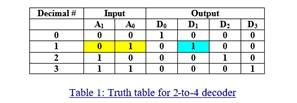As we see in the truth table (table 1), for each input combination, one output line is activated, that is, the output line corresponding to the input combination becomes 1, while other lines remain inactive. For example, an input of 00 at the input will activate line D0. 01 at the input will activate line D1, and so on.The circuit is implemented with AND gates, as shown in figure 3. In this circuit we see that the logic equation for D 0 is A1/ A0/. D0 is A1/ A0, and so on. These are in fact the minterms being implemented. Thus, each output of the decoder generates a minterm corresponding to the input combination.

# the enable input in decoder:

## -is one, then the decoder performs its normal operation.

For example, consider the 2-to-4 decoder with the enable input (Figure 4). The enable input is only responsible for making the decoder active or inactive. If Enable E is zero, then all outputs of the decoder will be zeros, regardless of the values of A 1 and A 0. However, if E is 1, then the decoder will perform its normal operation, as is shown in the

truth table (table 2). In this table we see that as long as E is zero, the outputs D0 to D3 will remain zero, no matter whatever value you provide at the inputs A1 A0, depicted by two don't cares. When E becomes 1, then we see the same behavior as we saw in the case of 2-to-4 decoder discussedearlier.##### Output waveforms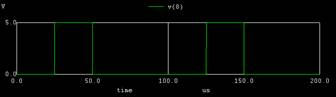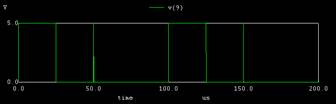COMPARISION OF DECODER WITH standard SN74LVC1G139 2-to-4 Line Decoder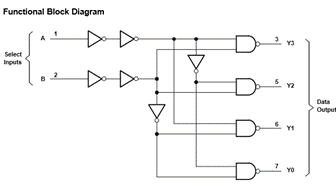of SN74LVC1G139 2-to-4 Line Decoder

DC ANAYSIS:

vdd 5 0 dc 5

v1 1 0 dc 5

v2 2 0 dc 5

.dc v1 0 5 01

.dc v2 0 5 01

OUTPUTS: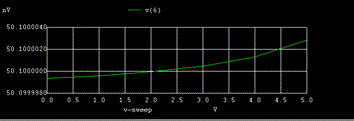#####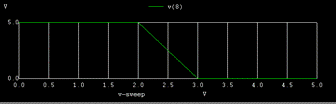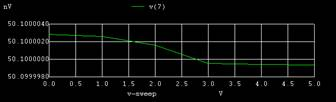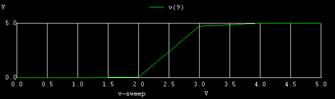whose inputs are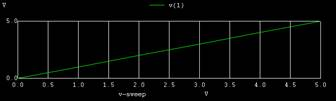this shows the theshold for 11 logic output at node 9 is high from the voltage 4.0 v similarly we can observe the thresholds for others output nodes.Here the standard version is providing a good range for logic 1.

When we compare with the components they provided the cicuit with extra two not gates which results in increment of chip size and the time delay for the output.

Time delay vs VCC for SN74LVC1G139 2-to-4 Line Decoder(TI):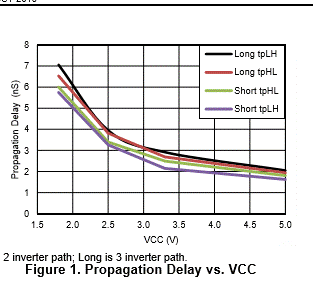whereas the outputs for the tpd for one observation is found to

be 4.99ns which is 2ns less .